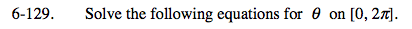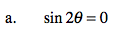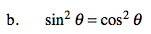### Home > PC > Chapter 6 > Lesson 6.3.2 > Problem6-129

6-129.
1. Solve the following equations for θ on [0, 2π]. Homework Help ✎

1. sin 2θ = 0

2. sin2 θ = cos2 θ1. Substitute using the Double-Angle Formula.
2. Set each factor equal to 0 and solve.1. Set the equation equal to 0.
2. Factor.
3. Set the factors equal to 0 and solve.
4. Note the domain.